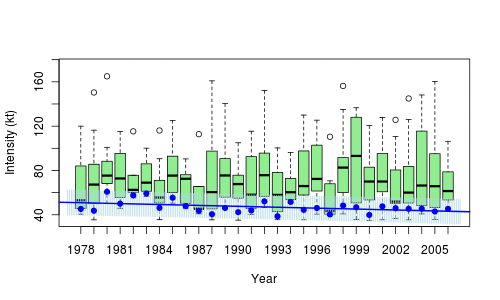Want to share your content on R-bloggers? click here if you have a blog, or here if you don't.This afternoon, I will be giving a two-hour talk at McGill on quantiles, quantile regressions, confidence regions, bagplots and outliers. Before defining (properly) quantile regressions, we will mention regression on (local) quantiles, as on the graph below, on hurricanes,In order to illustrate quantile regression, consider the following natality database,

```base=read.table(
"http://freakonometrics.free.fr/natality2005.txt",

We can use it produce those nice graphs we can find in several papers, modeling weight of newborns,

```u=seq(.05,.95,by=.01)
coefstd=function(u) summary(rq(WEIGHT~SEX+
SMOKER+WEIGHTGAIN+BIRTHRECORD+AGE+ BLACKM+
BLACKF+COLLEGE,data=base,tau=u))\$coefficients[,2]
coefest=function(u) summary(rq(WEIGHT~SEX+
SMOKER+WEIGHTGAIN+BIRTHRECORD+AGE+ BLACKM+
BLACKF+COLLEGE,data=base,tau=u))\$coefficients[,1]
CS=Vectorize(coefstd)(u)
CE=Vectorize(coefest)(u)```The slides can be downloaded on the blog, as well as the R-code.

Mcgill3

View more presentations from charthur.